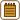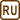## Atoms in the Iron Weight

### Task number: 1800

Imagine an iron weight with mass of 1kg. Determine:

a) What is the chemical amount of it?

b) How many atoms does it contain?

c) How many electrons does it contain and how many of them are valence?

d) What percentage of the mass of this iron weight is composed of electrons?

e) What volume corresponds to one atom?

f) If we imagine atoms as small cubes stacked next to each other, how great would be the edge of such a “monatomic cube”?

g) How long would be a row composed of these “cubes” if we stack them right next to each other? Compare the answer with a distance between two real places.

• #### Hint: The Amount of Substance

Due to the fact that the common amount of substance contains very high number of particles, we usually do not count them as separate pieces but in this case we use one of the fundamental units of measurement in the International System of Units (SI) for amount of substance – the mole. The respective physical quantity is called the amount of substance n (or chemical amount).

1 mole of substance means approximately 6.02·1023 particles. This number is represented by the so-called Avogadro constant, which is $$N_A\,\dot=\, 6{,}02 \cdot{10^{23}}\,\mathrm{mol^{-1}}$$. Therefore, we can determine the amount of substance n as the ratio of the total number of particles in the body N to the Avogadro constant NA.

$n\,=\,\frac{N}{N_A}\,.$
• #### Hint: Masses

In the Handbook of Chemistry and Physics we can find the so-called relative atomic mass Ar of the individual elements, which is a dimensionless number that is numerically equal to the mass of the body whose chemical amount is one mole, in grams. That means that for the molar mass Mm (= mass of 1 mole of particles) it holds that:

$M_m\,=\,A_r \,\cdot\, 1 \,\mathrm{\frac{g}{mol}}\,=\,A_r \,\cdot\, 10^{-3}\, \mathrm{\frac{kg}{mol}}\,.$

At the same time the relative atomic mass is equal to the ratio of the mass of one particle (e.g. atom) m0 to the atomic mass constant $$m_u\,\dot=\, 1.66 \cdot{10^{-27}}\,\mathrm{kg}.$$ Then

$A_r\,=\, \frac{m_0}{m_u}\hspace{20px} \Rightarrow\hspace{20px} m_0\,=\,A_r \,m_u\,.$

Double meaning of the relative atomic mass is given by the fact that for both constants mentioned above it holds that mu NA = 10-3 kg mol-1 (given by the definition of both constants).

• #### Definition and Comment on the Meaning of Constants

The atomic mass constant mu is defined as $$\frac{1}{12}$$ of the mass of an unbound atom of carbon $$^{12}_{\,\,6}\mathrm{C}$$ at rest and in its ground state . This atom consists of six neutrons, six protons and six electrons. Compared with the mass of proton and neutron, mass of electron is negligible. On the contrary, the masses of proton and neutron are very close. It implies that the atomic mass constant could be also represented as approximate mass of one nucleon (= proton or neutron) and then the relative atomic mass as the number of nucleons in the atom. Hence the mass of the atom m0 can be calculated as the product of the “number of nucleons” and “mass of single nucleon”.

Avogadro constant NA can be defined as the number of atoms in twelve grams of carbon nuclide $$^{12}_{\,\,6}\mathrm{C}$$. If you think through the definitions of both constants, you will easily substantiate their relationship mentioned in the previous section.

• #### a) Solution: The Amount of Substance

In the Handbook of Chemistry and Physics we will find the value for iron: Ar = 55.845

That means that the molar mass of iron is Mm = 55.845·10-3 kg mol-1.

We find out the amount of substance (= “amount of moles”) as the total mass of the iron weight m divided by the molar mass Mm:

$n\,=\,\frac{m}{M_m}\,=\,\frac{1 \mathrm{kg}}{55.845 \,\cdot\, 10^{-3}\,\mathrm{kg\,mol^{-1}}}\,\dot=\,17.9\,\mathrm{mol}$
• #### b) Solution: Number of Atoms

We can calculate the number of atoms N in the iron weight using two different methods. We can use calculated amount of substance n and Avogadro constant NA:

$N\,=\,n N_A\,=\,\frac{m N_A}{M_m}\,,$ $N\,=\,\frac{1 \mathrm{kg}\,\cdot\,6.022\,\cdot\,10^{23}\,\mathrm{mol^{-1}}}{55.845 \,\cdot\, 10^{-3}\,\mathrm{kg\,mol^{-1}}}\,,$ $N\,\dot=\,1.08 \,\cdot\,10^{25}$

or we use knowledge of the mass of one particle (from the relative atomic mass):

$N\,=\,\frac{m}{m_0}\,=\,\frac{m}{A_r m_u}\,,$ $N\,=\,\frac{1 \mathrm{kg}}{55.845 \,\cdot\,1.66\,\cdot\, 10^{-27}\,\mathrm{kg}}\,,$ $N\,\dot=\,1.08 \,\cdot\,10^{25}\,.$
• #### c) Solution: Number of Electrons

One atom of iron contains 26 electrons, from which 8 are valence (2 belong to the 4s2 subshell and 6 belong to the 3d6 subshell).

Total number of electrons:

$N_e\,=\,26N\,.$

We have calculated N in the previous section $$N\,\dot=\,1{,}08 \,\cdot\,10^{25}$$.

$N_e\,=\,26\,\cdot\, 1.08 \,\cdot\, 10^{25}\,=\, 2.8\,\cdot\, 10^{26}$

Total number of valence electrons:

$N_v\,=\,8N\,=\, 8\,\cdot\,1.08\,\cdot\,10^{25}\,=\, 8.6\,\cdot\,10^{25}$
• #### d) Solution: Mass of Electrons

Mass of one electron is me = 9.11·10-31 kg.

Mass of all electrons:

$m_\mathrm{{electron}}\,=\,N_e m_e$ $m_\mathrm{{electron}}\,=\,2.8\,\cdot\, 10^{26}\,\cdot\, 9.11\,\cdot\,10^{-31} \,\mathrm{kg}$ $m_\mathrm{{electron}}\,\dot=\, 2.6\,\cdot\, 10^{-4} \,\mathrm{kg}\,,$

which corresponds to 0.026% = 0.26‰.

Electrons contribute negligibly to the total atomic mass.

• #### e) Solution: Volume of One Atom

To determine the volume of one atom, we must first determine the volume of the entire iron weight using its density: ρ = 7 900 kg m-3.

$V\,=\,\frac{m}{\varrho}\,=\,\frac{1}{7\,900}\,\mathrm{m}^3$ $V\,\dot=\,1.27\,\cdot\,10^{-4}\,\mathrm{m}^3$

We obtain the volume of one atom V0 after dividing the total volume by the number of atoms:

$V_0\,=\,\frac{V}{N}\,\dot=\,\frac{1.27\,\cdot\, 10^{-4}}{1.08\,\cdot\, 10^{25}}\,\mathrm{m}^3\,,$ $V_0\,\dot=\,1.17\,\cdot\,10^{-29}\,\mathrm{m}^3\,.$

Or we can substitute N into the previous relation using its general expression and adjust the equation:

$V_0\,=\,\frac{V}{N}\,=\,\frac{m}{N \varrho}\,=\,\frac{m}{\frac{m N_A}{M_m}\,\varrho}\,=\,\frac{M_m}{\varrho\,N_A}$ $V_0\,\dot=\,\frac{55.845\,\cdot\, 10^{-3}}{6.022\,\cdot\, 10^{23}\,\cdot\,7900}\,\mathrm{m}^3$ $V_0\,\dot=\,1.17\,\cdot\, 10^{-29}\,\mathrm{m}^3$
• #### f) and g) Solution: Edge of the Cube and Length of the Line

f) For the volume of cube V it holds that: V = a3, where a is the length of its edge.

Length of the edge of the imaginary “monatomic cube” thus will be:

$a\,=\, \sqrt{V_0}\dot=\,\sqrt{1.17\,\cdot\,10^{-29}}\,\mathrm{m}\,,$ $a\,\dot=\,2.27\,\cdot\,10^{-10}\,\mathrm{m}\,.$

g) If we stack these cubes in line, the total length of the line would be:

$l\,=\, N \cdot\,a\,\dot=\,1.08\,\cdot\,10^{25}\,\cdot\, 2.27\,\cdot\,10^{-10}\,\mathrm{m}\,,$ $l\,\dot=\,2.45\,\cdot\,10^{15}\,\mathrm{m}\,.$

This distance corresponds approximately to one fourth of a light year.

• #### Answer

In the one-kilogram iron weight there are 1.08·1025 atoms, which corresponds to the chemical amount of approximately 17.9 moles of atoms. In total there are 2.8·1026 electrons and 8.6·1025 of them are valence.

Mass of all electrons is about 0.26 g, i.e. electrons contribute very slightly to the mass of the entire weight.

The volume of one single atom is about 1.17·10-29 m3, i.e. cube with the edge of approximately 0.227 nm. If we stack these cubes in line, the length of the line would be about a quarter of the light year.